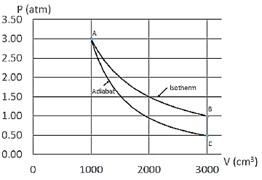# Problem: An ideal gas is taken through the cycle shown in the diagram below. The process is carried out with 150 mg of Helium, which is a monoatomic gas with an atomic mass of 4 g/mol. First, the gas undergoes an adiabatic expansion (from point A to point C), followed by an increase in pressure at constant volume (from point C to point B). Finally, the gas is taken back to the initial state by an isothermal compression (from point B to point A). What is the change in entropy of the gas during the isothermal process (from point B to point A)? Note that the work during an isothermal process is W = nRT ln(Vi / Vf).

83% (57 ratings)
###### Problem Details

An ideal gas is taken through the cycle shown in the diagram below. The process is carried out with 150 mg of Helium, which is a monoatomic gas with an atomic mass of 4 g/mol. First, the gas undergoes an adiabatic expansion (from point A to point C), followed by an increase in pressure at constant volume (from point C to point B). Finally, the gas is taken back to the initial state by an isothermal compression (from point B to point A). What is the change in entropy of the gas during the isothermal process (from point B to point A)? Note that the work during an isothermal process is W = nRT ln(Vi / Vf).Frequently Asked Questions

What scientific concept do you need to know in order to solve this problem?

Our tutors have indicated that to solve this problem you will need to apply the Entropy and the Second Law concept. You can view video lessons to learn Entropy and the Second Law. Or if you need more Entropy and the Second Law practice, you can also practice Entropy and the Second Law practice problems.

How long does this problem take to solve?

Our expert Physics tutor, Jeffery took 4 minutes and 28 seconds to solve this problem. You can follow their steps in the video explanation above.